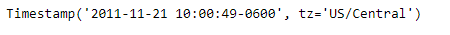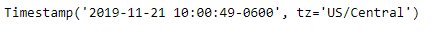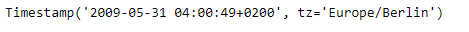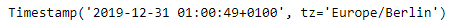Related Articles

# Python | Pandas Timestamp.replace

• Last Updated : 14 Jan, 2019

Python is a great language for doing data analysis, primarily because of the fantastic ecosystem of data-centric python packages. Pandas is one of those packages and makes importing and analyzing data much easier.

Pandas` Timestamp.replace()` function is used to replace the member values of the given Timestamp. The function implements datetime.replace, and it also handles nanoseconds.

Syntax :Timestamp.replace()

Parameters :
year : int
month : int
day : int
hour : int
minute : int
second : int
microsecond : int
nanosecond : int
tzinfo : int
fold : int

Return : Timestamp with fields replaced

Example #1: Use `Timestamp.replace()` function to replace the year value in the given Timestamp.

 `# importing pandas as pd``import` `pandas as pd`` ` `# Create the Timestamp object``ts ``=` `pd.Timestamp(year ``=` `2011``,  month ``=` `11``, day ``=` `21``,``                  ``hour ``=` `10``, second ``=` `49``, tz ``=` `'US/Central'``)`` ` `# Print the Timestamp object``print``(ts)`

Output :Now we will use the `Timestamp.replace()` function to replace the current year in the object with 2019.

 `# replace year``ts.replace(year ``=` `2019``)`

Output :As we can see in the output, the `Timestamp.replace()` function has returned a Timestamp object with year value equal to 2019.

Example #2: Use `Timestamp.replace()` function to replace the year, month and hour value in the given Timestamp.

 `# importing pandas as pd``import` `pandas as pd`` ` `# Create the Timestamp object``ts ``=` `pd.Timestamp(year ``=` `2009``, month ``=` `5``, day ``=` `31``,``                  ``hour ``=` `4``, second ``=` `49``, tz ``=` `'Europe/Berlin'``)`` ` `# Print the Timestamp object``print``(ts)`

Output :Now we will use the `Timestamp.replace()` function to replace the current year, month and hour value in the object.

 `# replace year, month and hour value``ts.replace(year ``=` `2019``, month ``=` `12``, hour ``=` `1``)`

Output :As we can see in the output, the `Timestamp.replace()` function has returned a Timestamp object with year value equal to 2019, month value equal to 12 and hour value equal to 1.

Attention geek! Strengthen your foundations with the Python Programming Foundation Course and learn the basics.

To begin with, your interview preparations Enhance your Data Structures concepts with the Python DS Course. And to begin with your Machine Learning Journey, join the Machine Learning – Basic Level Course

My Personal Notes arrow_drop_up